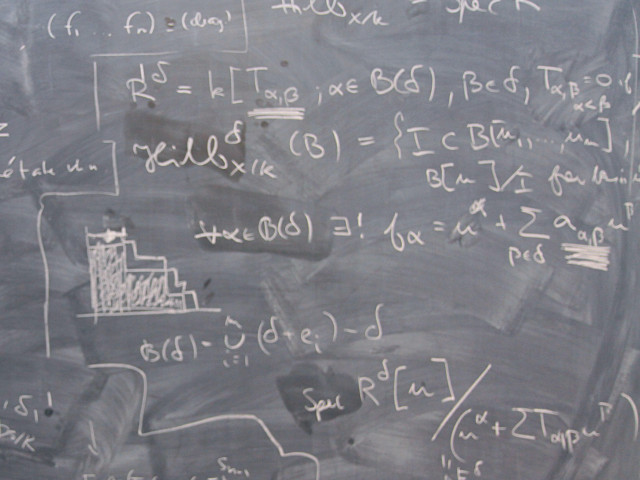SF1637 Differential Equations and Transforms III 6.0 creditsBasic course in differential equations and Fourier analysis.

Course offering missing for current semester as well as for previous and coming semesters
Headings with content from the Course syllabus SF1637 (Autumn 2007–) are denoted with an asterisk ( )

Content and learning outcomes

Course contents

• First order ordinary differential equations: Basic theory and concepts. Modelling. Direction fields and solution curves. Autonomous equations, stationary solutions and stability. Separable equations. Linear equations. Substitutions.
• Linear ordinary differential equations of higher order: Basic theory. Methods for solving equations with constant coefficients. The harmonic oscillator.
• Systems of linear ordinary differential equations: Basic theory and concepts. Solutions to linear systems with constant coefficients using the eigenvalue method (homogeneous systems) and ”variation of parameters” (particular solutions to non-homogeneous systems).
• Autonomous systems of ordinary differential equations: Basic concepts. Stationary solutions and stability. Brief discussion about global phase portraits. Modelling
• Fourier series and Fourier transforms with applications.
• Linear partial differential equations: Separation of variables. Solutions to classical boundary value problems (the wave equation, the heat equation, Laplace’s equation) using Fourier methods.
• Program specific contents.

Intended learning outcomes

To provide the students with

• Knowledge of basic theory of ordinary differential equations
• Ability to solve certain types of (systems of) ordinary differential equations with standard methods
• Ability to investigate (systems of) ordinary differential equations with elementary geometrical and qualitative methods
• Ability to compute Fourier series and Fourier transforms
• Ability to solve separable partial differential equations and to find solutions to boundary value problems using Fourier methods.
• Possibilities of further studies of related topics specific to their educational programme
• Ability to use suitable software for symbolic as well as graphical investigations of the types of problems mentioned above
• Ability to apply these skills to mathematical modelling.

Course disposition

No information inserted

Literature and preparations

Specific prerequisites

Basic knowledge in linear algebra and calculus equivalent to 5B1143/SF1623 Mathematics 1 for teachers and 5B1123/SF1613 Mathematics 2 for teachers, or 5B1104/SF1600 + 5B1105/SF1601 Calculus I, part 1 + 2 and 5B1109/SF1604 Linear algebra.

Recommended prerequisites

No information inserted

Equipment

No information inserted

Literature

Zill-Cullen/Differential Equations with Boundary-Value Problems, 6:th ed.

Examination and completion

If the course is discontinued, students may request to be examined during the following two academic years.

A, B, C, D, E, FX, F

Examination

• TEN1 - Examination, 6.0 credits, grading scale: A, B, C, D, E, FX, F

Based on recommendation from KTH’s coordinator for disabilities, the examiner will decide how to adapt an examination for students with documented disability.

The examiner may apply another examination format when re-examining individual students.

TEN1, 6 hp. Final oral and/or written exam and continuous examination.

Opportunity to complete the requirements via supplementary examination

No information inserted

Opportunity to raise an approved grade via renewed examination

No information inserted

Examiner

No information inserted

Ethical approach

• All members of a group are responsible for the group's work.
• In any assessment, every student shall honestly disclose any help received and sources used.
• In an oral assessment, every student shall be able to present and answer questions about the entire assignment and solution.

Further information

Course web

Further information about the course can be found on the Course web at the link below. Information on the Course web will later be moved to this site.

Course web SF1637

SCI/Mathematics

Main field of study

Mathematics, Technology

First cycle